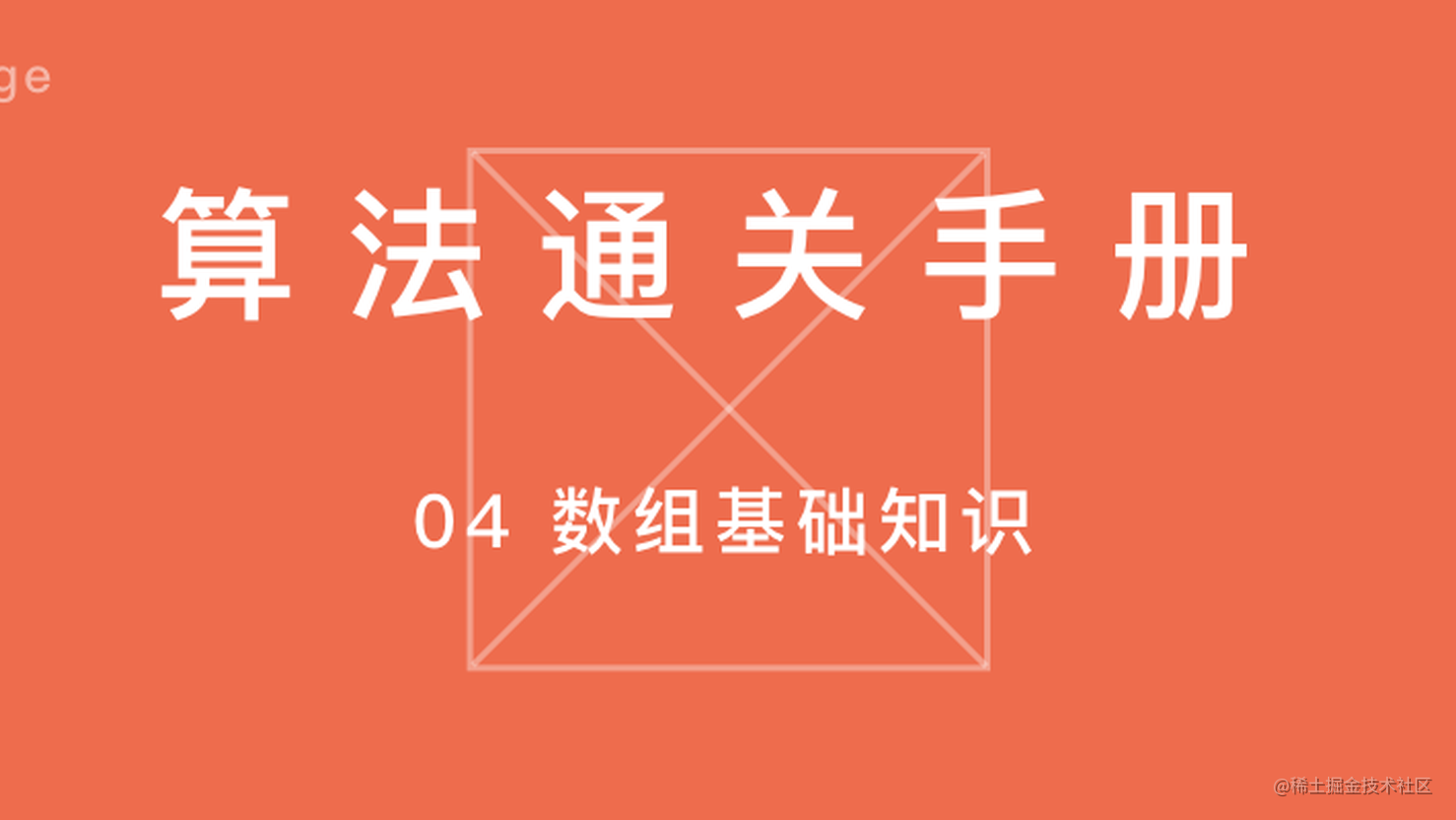# 算法通关手册：04 数组基础知识

## 1. 数组简介

### 1.1 数组定义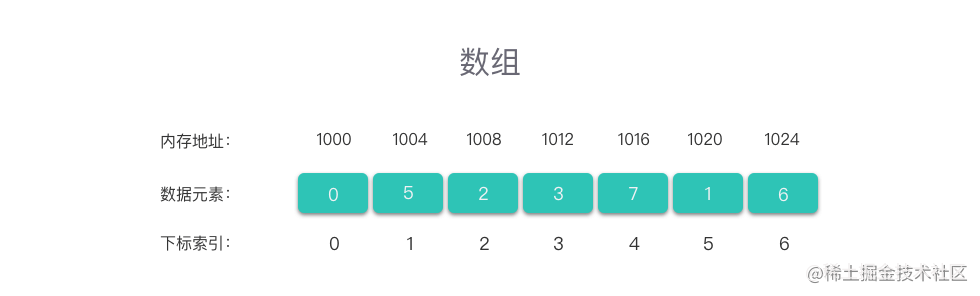• 第一个方面是 「线性表」

• 第二个方面是 「连续的内存空间」

### 1.3 多维数组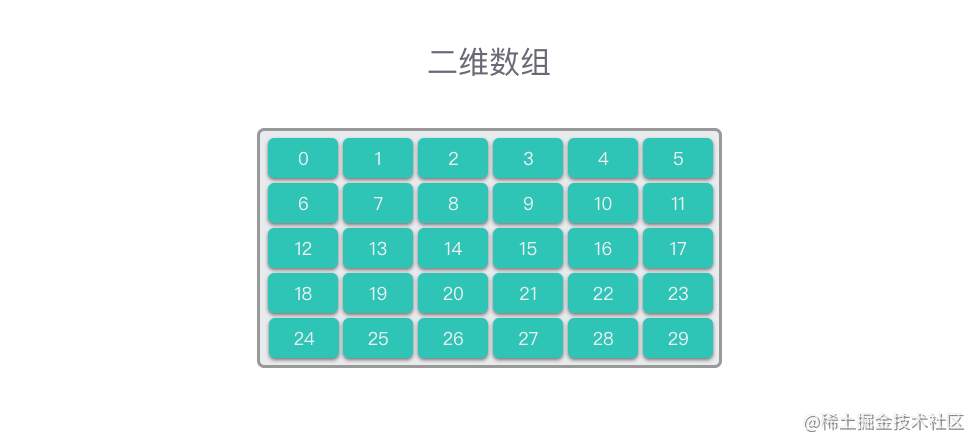### 1.4 不同编程语言中数组的实现

C / C++ 中的数组最接近数组结构定义中的数组，使用的是一块存储相同类型数据的、连续的内存空间。不管是基本类型数据，还是结构体、对象，在数组中都是连续存储的。例如：int arr = {{0, 1, 2, 3}, {4, 5, 6, 7}, {8, 9, 10, 11}};

Java 中的数组跟数据结构定义中的数组不太一样。 Java 中的数组也是存储相同类型数据的，但所使用的内存空间却不一定是连续（多维数组中）。且如果是多维数组，其嵌套数组的长度也可以不同。例如：int[][] arr = new int[]{ {1,2,3}, {4,5}, {6,7,8,9}};

## 2. 数组的基本操作

### 2.1 访问元素

# 从数组 nums 中读取下标为 i 的数据元素值
def value(nums, i):
if 0 <= i <= len(nums) - 1:
print(nums[i])

arr = [0, 5, 2, 3, 7, 1, 6]
value(arr, 3)

### 2.2 查找元素

# 从数组 nums 中查找元素值为 val 的数据元素第一次出现的位置
def find(nums, val):
for i in range(len(nums)):
if nums[i] == val:
return i
return -1

arr = [0, 5, 2, 3, 7, 1, 6]
print(find(arr, 5))

### 2.3 插入元素

Python 中的 list 直接封装了尾部插入操作，直接调用 append 方法即可。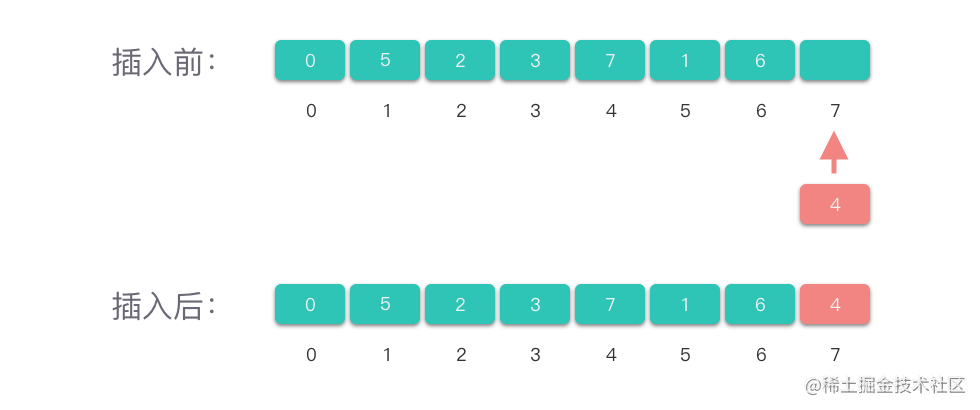arr = [0, 5, 2, 3, 7, 1, 6]
val = 4
arr.append(val)
print(arr)

Python 中的 list 直接封装了中间插入操作，直接调用 insert 方法即可。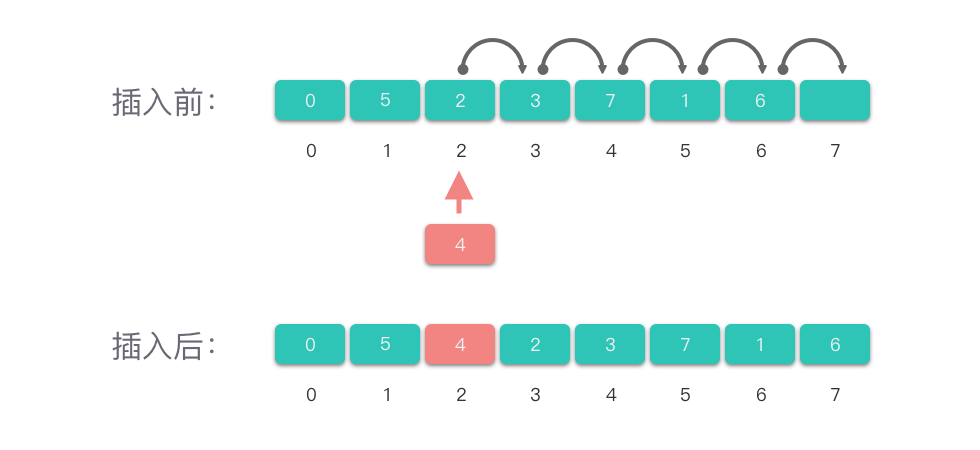arr = [0, 5, 2, 3, 7, 1, 6]
i, val = 2, 4
arr.insert(i, val)
print(arr)

### 2.4 改变元素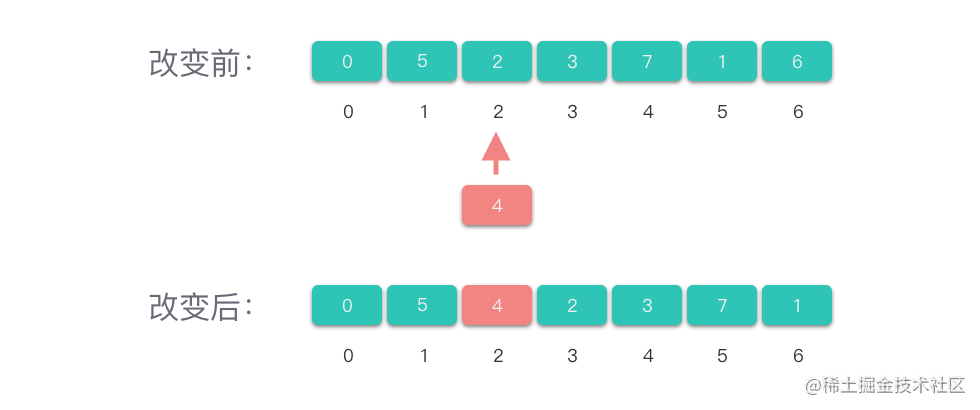def change(nums, i, val):
if 0 <= i <= len(nums) - 1:
nums[i] = val

arr = [0, 5, 2, 3, 7, 1, 6]
i, val = 2, 4
change(arr, i, val)
print(arr)

### 2.5 删除元素

Python 中的 list 直接封装了删除数组尾部元素的操作，只需要调用 pop 方法即可。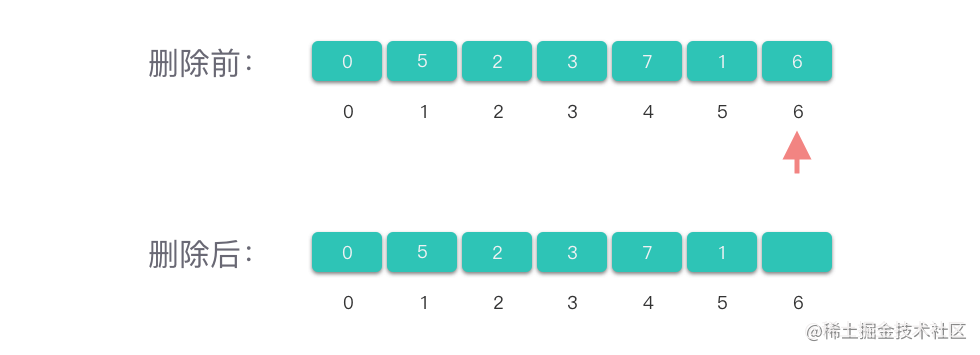arr = [0, 5, 2, 3, 7, 1, 6]
arr.pop()
print(arr)

Python 中的 list 直接封装了删除数组中间元素的操作，只需要以下标作为参数调用 pop 方法即可。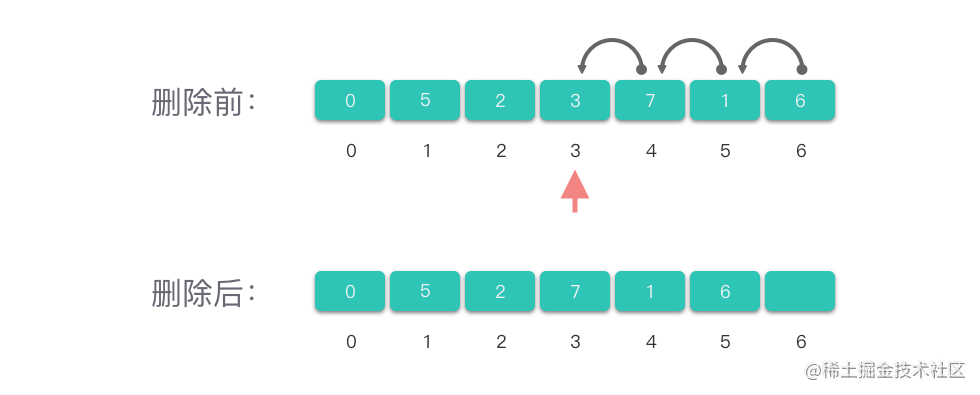arr = [0, 5, 2, 3, 7, 1, 6]
i = 3
arr.pop(i)
print(arr)

arr = [0, 5, 2, 3, 7, 1, 6]
i = 3
arr.remove(5)
print(arr)